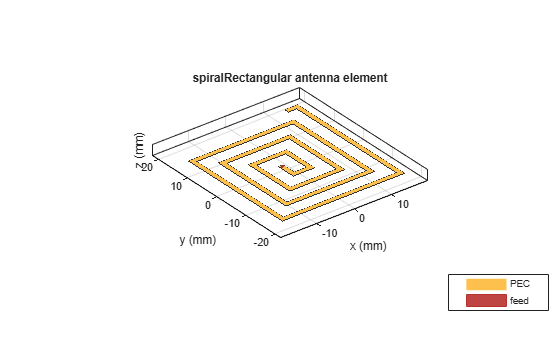# rectspirallength2turns

Calculate number of turns for specified arm length in rectangular spiral antenna

## Syntax

``Nturns = rectspirallength2turns(ant,reqtotalarmlength)``

## Description

example

````Nturns = rectspirallength2turns(ant,reqtotalarmlength)` calculates the equivalent number of turns for a specified total arm length in a rectangular spiral antenna.```

## Examples

collapse all

Create a single arm rectangular spiral antenna with a total arm length of 291 mm.

```ant = spiralRectangular('NumArms',1,'NumTurns',3,'InitialLength',4.5e-3,... 'InitialWidth',4.5e-3,'Spacing',3.3e-3,'StripWidth',1.2e-3); nT = rectspirallength2turns(ant,291e-3); ant.NumTurns = nT; figure; show(ant); ```## Input Arguments

collapse all

Rectangular spiral antenna, specified as a `spiralRectangular` object.

Total length of the rectangular spiral antenna arm, specified as a scalar in meters. In case of dual arm, the input takes the length of any one of the arms.

Example: 33e-3

## Output Arguments

collapse all

Equivalent number of turns for a specified total arm length, returned as a scalar.

## Version History

Introduced in R2020a